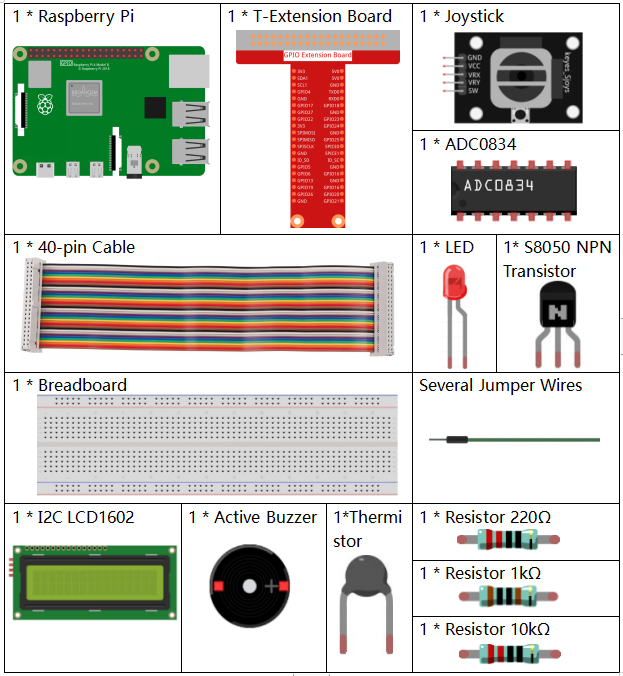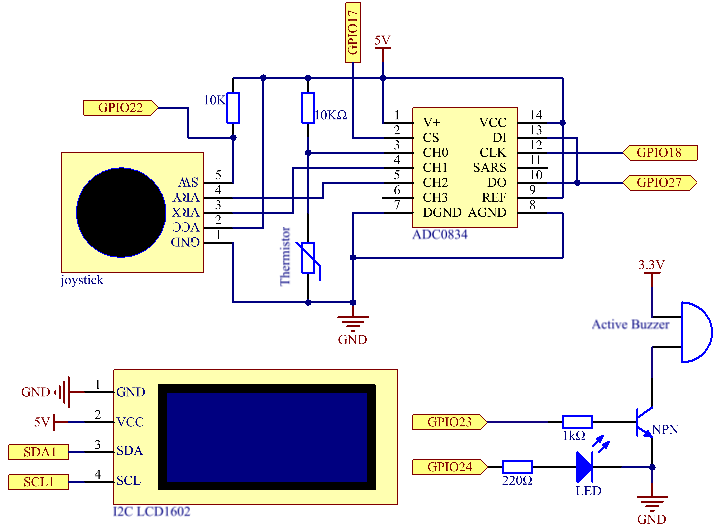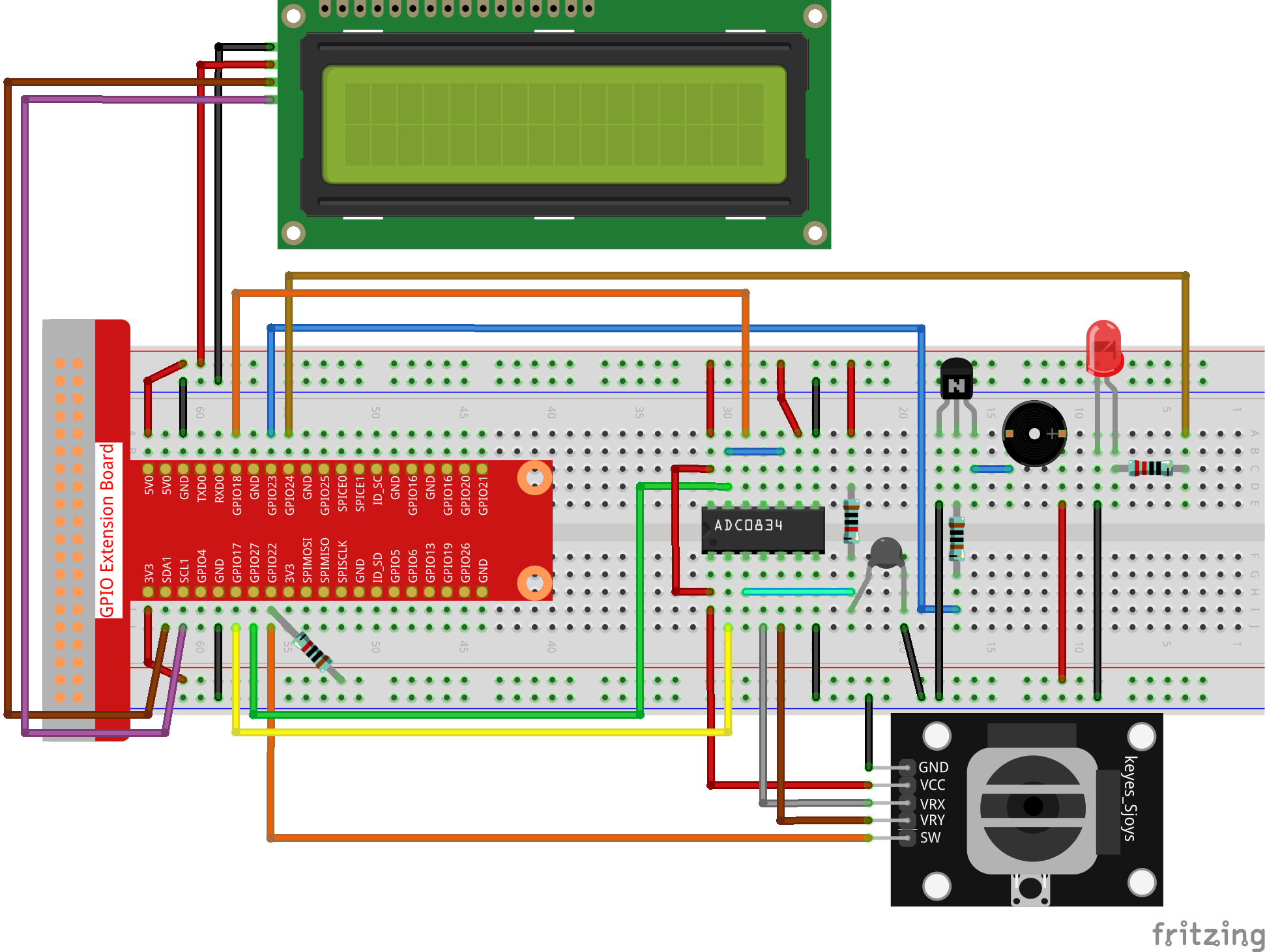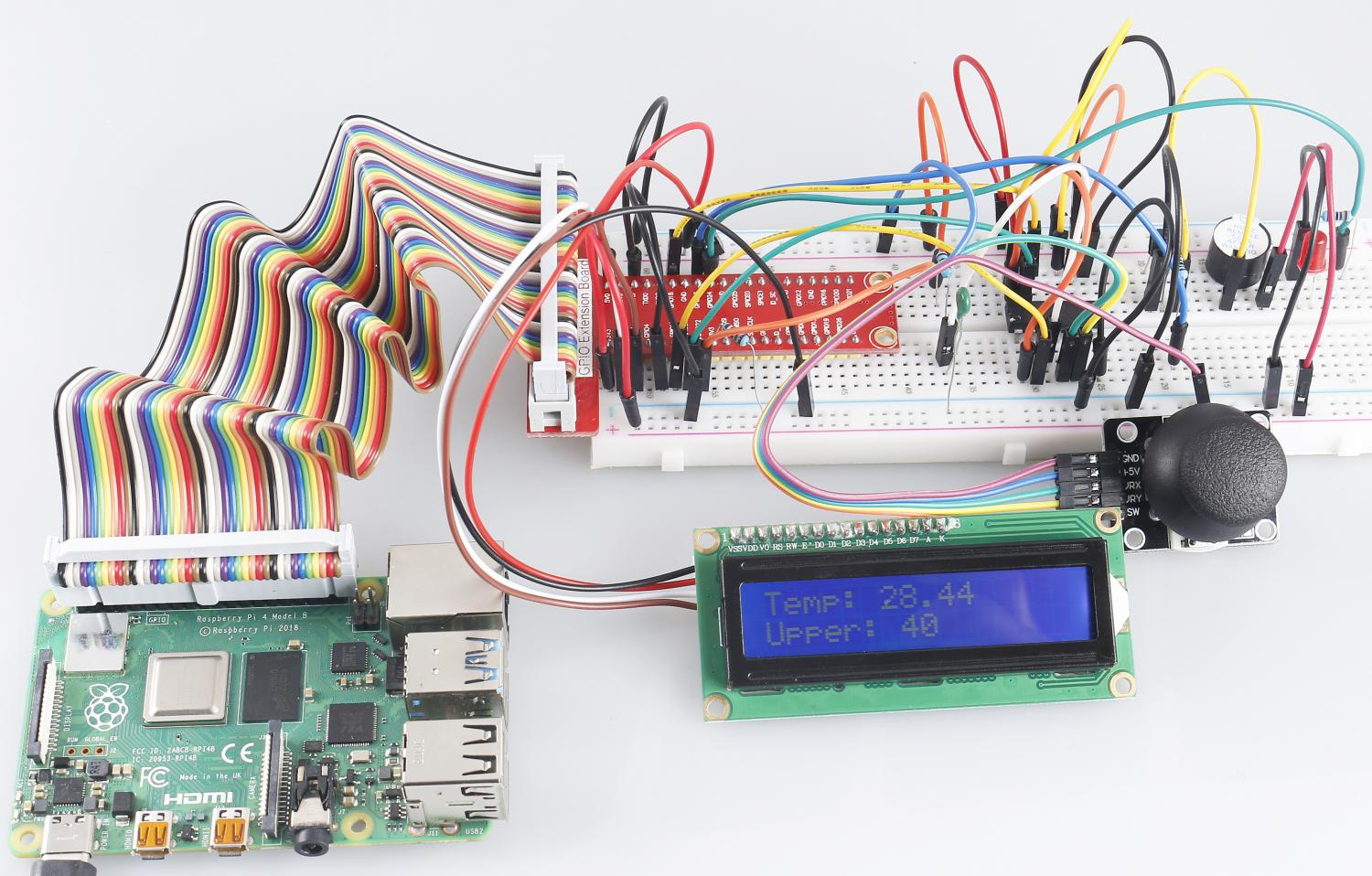# 4.1.13 Overheat Monitor¶

## Introduction¶

You may want to make an overheat monitoring device that applies to various situations, ex., in the factory, if we want to have an alarm and the timely automatic turning off of the machine when there is a circuit overheating. In this project, we will use thermistor, joystick, buzzer, LED and LCD to make an smart temperature monitoring device whose threshold is adjustable.

## Components¶## Schematic Diagram¶

 T-Board Name physical wiringPi BCM GPIO17 Pin 11 0 17 GPIO18 Pin 12 1 18 GPIO27 Pin 13 2 27 GPIO22 Pin15 3 22 GPIO23 Pin16 4 23 GPIO24 Pin18 5 24 SDA1 Pin 3 SCL1 Pin 5## Experimental Procedures¶

Step 1: Build the circuit.Step 2: Go to the folder of the code.

```cd /home/pi/raphael-kit/python/
```

Step 3: Run the executable file.

```sudo python3 4.1.13_OverheatMonitor.py
```

As the code runs, the current temperature and the high-temperature threshold 40 are displayed on I2C LCD1602. If the current temperature is larger than the threshold, the buzzer and LED are started to alarm you.

Joystick here is for your pressing to adjust the high-temperature threshold. Toggling the Joystick in the direction of X-axis and Y-axis can adjust (turn up or down) the current high-temperature threshold. Press the Joystick once again to reset the threshold to initial value.

Code

Note

You can Modify/Reset/Copy/Run/Stop the code below. But before that, you need to go to source code path like `raphael-kit/python`. After modifying the code, you can run it directly to see the effect.

```#!/usr/bin/env python3

import LCD1602
import RPi.GPIO as GPIO
import time
import math

Joy_BtnPin = 22
buzzPin = 23
ledPin = 24

upperTem = 40

def setup():
GPIO.setmode(GPIO.BCM)
GPIO.setup(ledPin, GPIO.OUT, initial=GPIO.LOW)
GPIO.setup(buzzPin, GPIO.OUT, initial=GPIO.LOW)
GPIO.setup(Joy_BtnPin, GPIO.IN, pull_up_down=GPIO.PUD_UP)
LCD1602.init(0x27, 1)

def get_joystick_value():
if(x_val > 200):
return 1
elif(x_val < 50):
return -1
elif(y_val > 200):
return -10
elif(y_val < 50):
return 10
else:
return 0

def upper_tem_setting():
global upperTem
LCD1602.write(0, 0, 'Upper Adjust: ')
change = int(get_joystick_value())
upperTem = upperTem + change
strUpperTem = str(upperTem)
LCD1602.write(0, 1, strUpperTem)
LCD1602.write(len(strUpperTem),1, '              ')
time.sleep(0.1)

def temperature():
Vr = 5 * float(analogVal) / 255
Rt = 10000 * Vr / (5 - Vr)
temp = 1/(((math.log(Rt / 10000)) / 3950) + (1 / (273.15+25)))
Cel = temp - 273.15
Fah = Cel * 1.8 + 32
return round(Cel,2)

def monitoring_temp():
global upperTem
Cel=temperature()
LCD1602.write(0, 0, 'Temp: ')
LCD1602.write(0, 1, 'Upper: ')
LCD1602.write(6, 0, str(Cel))
LCD1602.write(7, 1, str(upperTem))
time.sleep(0.1)
if Cel >= upperTem:
GPIO.output(buzzPin, GPIO.HIGH)
GPIO.output(ledPin, GPIO.HIGH)
else:
GPIO.output(buzzPin, GPIO.LOW)
GPIO.output(ledPin, GPIO.LOW)

def loop():
lastState=1
stage=0
while True:
currentState=GPIO.input(Joy_BtnPin)
if currentState==1 and lastState ==0:
stage=(stage+1)%2
time.sleep(0.1)
LCD1602.clear()
lastState=currentState
if stage == 1:
upper_tem_setting()
else:
monitoring_temp()

def destroy():
LCD1602.clear()
GPIO.cleanup()

if __name__ == '__main__':     # Program start from here
try:
setup()
while True:
loop()
except KeyboardInterrupt:   # When 'Ctrl+C' is pressed, the program destroy() will be executed.
destroy()
```

Code Explanation

```def get_joystick_value():
if(x_val > 200):
return 1
elif(x_val < 50):
return -1
elif(y_val > 200):
return -10
elif(y_val < 50):
return 10
else:
return 0
```

This function reads values of X and Y. If X>200, there will return “1”; X<50, return “-1”; y>200, return “-10”, and y<50, return “10”.

```def upper_tem_setting():
global upperTem
LCD1602.write(0, 0, 'Upper Adjust: ')
change = int(get_joystick_value())
upperTem = upperTem + change
LCD1602.write(0, 1, str(upperTem))
LCD1602.write(len(strUpperTem),1, '              ')
time.sleep(0.1)
```

This function is for adjusting the threshold and displaying it on the I2C LCD1602.

```def temperature():
Vr = 5 * float(analogVal) / 255
Rt = 10000 * Vr / (5 - Vr)
temp = 1/(((math.log(Rt / 10000)) / 3950) + (1 / (273.15+25)))
Cel = temp - 273.15
Fah = Cel * 1.8 + 32
return round(Cel,2)
```

Read the analog value of the CH0 (thermistor) of ADC0834 and then convert it to temperature value.

```def monitoring_temp():
global upperTem
Cel=temperature()
LCD1602.write(0, 0, 'Temp: ')
LCD1602.write(0, 1, 'Upper: ')
LCD1602.write(6, 0, str(Cel))
LCD1602.write(7, 1, str(upperTem))
time.sleep(0.1)
if Cel >= upperTem:
GPIO.output(buzzPin, GPIO.HIGH)
GPIO.output(ledPin, GPIO.HIGH)
else:
GPIO.output(buzzPin, GPIO.LOW)
GPIO.output(ledPin, GPIO.LOW)
```

As the code runs, the current temperature and the high-temperature threshold 40 are displayed on I2C LCD1602. If the current temperature is larger than the threshold, the buzzer and LED are started to alarm you.

```def loop():
lastState=1
stage=0
while True:
currentState=GPIO.input(Joy_BtnPin)
if currentState==1 and lastState ==0:
stage=(stage+1)%2
time.sleep(0.1)
LCD1602.clear()
lastState=currentState
if stage == 1:
upper_tem_setting()
else:
monitoring_temp()
```

The function `main()` contains the whole program process as shown:

1. When the program starts, the initial value of stage is 0, and the current temperature and the high-temperature threshold 40 are displayed on I2C LCD1602. If the current temperature is larger than the threshold, the buzzer and the LED are started to alarm you.

2. Press the Joystick, and stage will be 1 and you can adjust the high-temperature threshold. Toggling the Joystick in the direction of X-axis and Y-axis can adjust (turn up or down) the current high-temperature threshold. Press the Joystick once again to reset the threshold to initial value.

## Phenomenon Picture¶